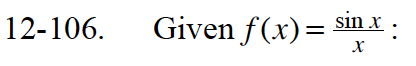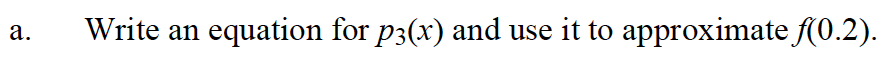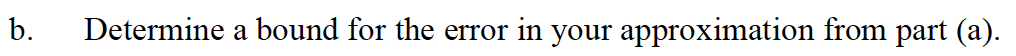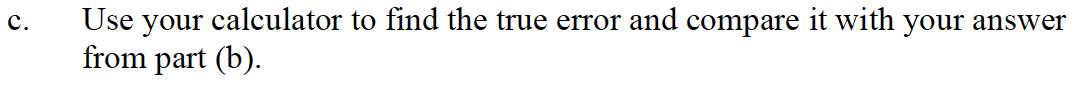### Home > CALC > Chapter 12 > Lesson 12.3.1 > Problem12-106

12-106.
1. Given f(x) =: Homework Help ✎

1. Write an equation for p3(x) and use it to approximate f(0.2).

2. Determine a bound for the error in your approximation from part (a).

3. Use your calculator to find the true error and compare it with your answer from part (b).The next non-zero term in the approximation is: